This is an analysis of some 911 call data from Kaggle that I took as a progress milestone to cover for the first batch of learnings.

## Data and Setup

``````import numpy as np
import pandas as pd
import matplotlib.pyplot as plt
import seaborn as sns
%matplotlib inline
``````

### Getting started - Data Access

``````df = pd.read_csv('911.csv')

``````
lat lng desc zip title timeStamp twp addr e
0 40.297876 -75.581294 REINDEER CT & DEAD END; NEW HANOVER; Station ... 19525.0 EMS: BACK PAINS/INJURY 2015-12-10 17:40:00 NEW HANOVER REINDEER CT & DEAD END 1
1 40.258061 -75.264680 BRIAR PATH & WHITEMARSH LN; HATFIELD TOWNSHIP... 19446.0 EMS: DIABETIC EMERGENCY 2015-12-10 17:40:00 HATFIELD TOWNSHIP BRIAR PATH & WHITEMARSH LN 1
2 40.121182 -75.351975 HAWS AVE; NORRISTOWN; 2015-12-10 @ 14:39:21-St... 19401.0 Fire: GAS-ODOR/LEAK 2015-12-10 17:40:00 NORRISTOWN HAWS AVE 1
``````df.info()
``````
``````<class 'pandas.core.frame.DataFrame'>
RangeIndex: 99492 entries, 0 to 99491
Data columns (total 9 columns):
lat          99492 non-null float64
lng          99492 non-null float64
desc         99492 non-null object
zip          86637 non-null float64
title        99492 non-null object
timeStamp    99492 non-null object
twp          99449 non-null object
e            99492 non-null int64
dtypes: float64(3), int64(1), object(5)
memory usage: 6.8+ MB
``````

### Top 5 zipcodes for 911 calls

``````df['zip'].value_counts().head(5)
``````
``````19401.0    6979
19464.0    6643
19403.0    4854
19446.0    4748
19406.0    3174
Name: zip, dtype: int64
``````

### Top 5 townships (twp) for 911 calls

``````df['twp'].value_counts().head(5)
``````
``````LOWER MERION    8443
ABINGTON        5977
NORRISTOWN      5890
UPPER MERION    5227
CHELTENHAM      4575
Name: twp, dtype: int64
``````

### How many unique ‘title’ codes are there?

``````len(df['title'].value_counts())
``````
``````110
``````

### Creating new features

In the titles column there are “Reasons/Departments” specified before the title code.

These are EMS, Fire, and Traffic.

Use .apply() with a custom lambda expression to create a new column called “Reason” that contains this string value.

For example, if the title column value is EMS: BACK PAINS/INJURY , the Reason column value would be EMS.

``````df['Reason'] = df['title'].apply(lambda title: title.split(':'))

``````
``````0     EMS
1     EMS
2    Fire
3     EMS
4     EMS
Name: Reason, dtype: object
``````

### The most common Reason for a 911 call based off of this new column

``````df['Reason'].value_counts()
``````
``````EMS        48877
Traffic    35695
Fire       14920
Name: Reason, dtype: int64
``````

### Use Seaborn to Create a countplot of 911 calls by Reason.

``````sns.countplot(x='Reason', data= df, palette='coolwarm')
``````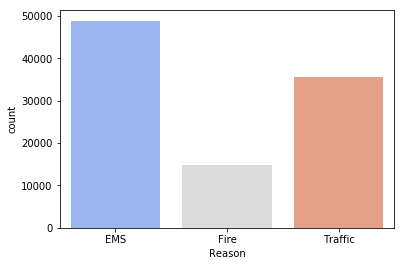## Focus on time information.

Data type of the objects in the timeStamp column

``````type(df['timeStamp'])
``````
``````pandas._libs.tslib.Timestamp
``````

### convert the ‘timeStamp’ column from strings to DateTime objects

``````df['timeStamp'] = pd.to_datetime(df['timeStamp'])

type(df['timeStamp'])
``````
``````pandas._libs.tslib.Timestamp
``````
``````df['timeStamp']
``````
``````Timestamp('2015-12-10 17:40:01')
``````

### Extract time components eg. Month, hour

``````print(df['timeStamp'].month)
print(df['timeStamp'].hour)
``````
``````12
17
``````

### Create 3 new columns called Hour, Month, and Day_of_Week

``````df['Month'] = df['timeStamp'].apply(lambda timestamp: timestamp.month)

df['Hour'] = df['timeStamp'].apply(lambda timestamp: timestamp.hour)

df['Day_of_week'] = df['timeStamp'].apply(lambda timestamp: timestamp.weekday())

``````

#### Use the .map() with this dictionary to map the actual string names to the day of the week:

``````dmap = {0:'Mon',1:'Tue',2:'Wed',3:'Thu',4:'Fri',5:'Sat',6:'Sun'}

df['Day_of_week'] = df['timeStamp'].apply(lambda timestamp: timestamp.weekday()).map(dmap)

``````

## With Seaborn create:

### Countplot of the Day of Week with the hue based off of the Reason

``````sns.countplot(data=df, x='Day_of_week',hue='Reason')
plt.legend(loc='center left', bbox_to_anchor=(1.0, 0.5))
``````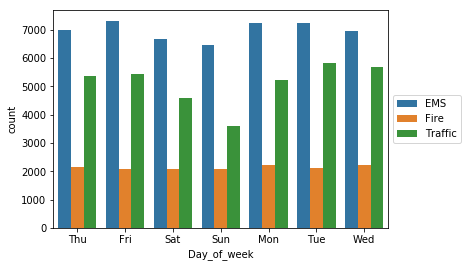### Now Countplot for Month:

``````sns.countplot(data=df, x='Month',hue='Reason')
plt.legend(loc='center left', bbox_to_anchor=(1.0, 0.5))
``````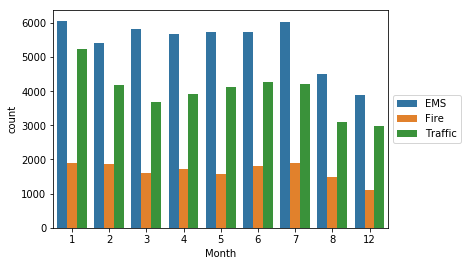The dataset is missing some Months,

fill in this information by possibly a simple line plot that fills in the missing months.

Now create a gropuby object called byMonth, where you group the DataFrame by the month column and use the count() method for aggregation.

``````byMonth = df.groupby('Month').count()
``````

#### A simple plot of the DataFrame indicating the count of calls per month.

``````byMonth['twp'].plot()
``````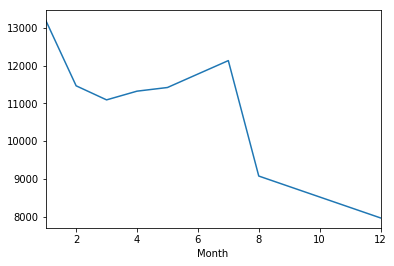### Create a linear fit on the number of calls per month.

Keep in mind you may need to reset the index to a column.

``````sns.lmplot(data=byMonth.reset_index(), x='Month',y='twp')
``````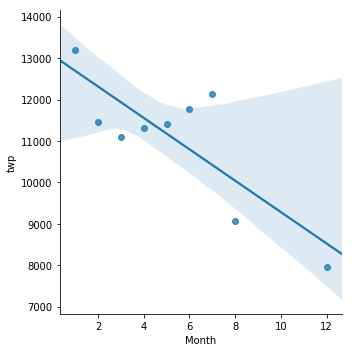Create a new column called ‘Date’ that contains the date from the timeStamp column.

You’ll need to use apply along with the .date() method.

``````df['Date'] = df['timeStamp'].apply(lambda timestamp: timestamp.date())
``````

Group by this Date column with the count() aggregate

and create a plot of counts of 911 calls.

``````df.groupby('Date').count()['twp'].plot()
plt.tight_layout()
``````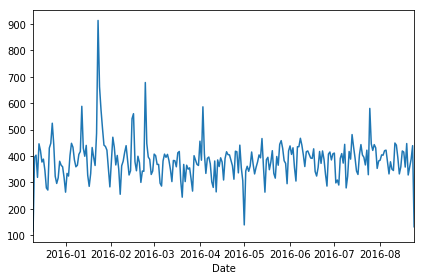## Create plots representing a Reason for the 911 call

#### Plot representing EMS calls

``````df[df['Reason']=='EMS'].groupby('Date').count()['twp'].plot()
plt.tight_layout()
plt.title('EMS')
``````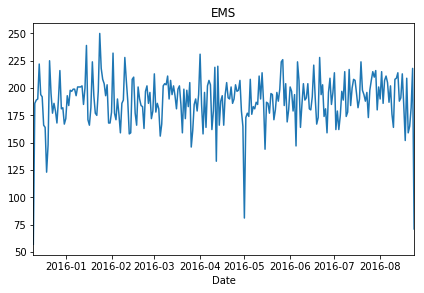#### Plot representing Fire calls

``````df[df['Reason']=='Fire'].groupby('Date').count()['twp'].plot()
plt.tight_layout()
plt.title('Fire')
``````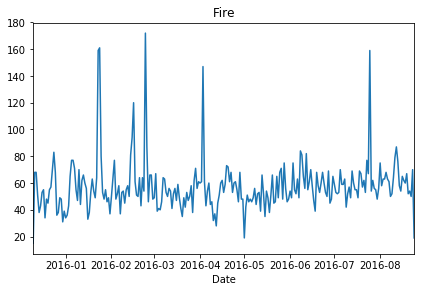#### Plot representing Traffic calls

``````df[df['Reason']=='Traffic'].groupby('Date').count()['twp'].plot()
plt.tight_layout()
plt.title('Traffic')
``````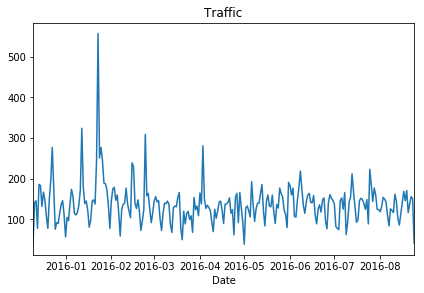## Creating Heatmaps with Seaborn

Restructure the dataframe so that the columns become the Hours, Index becomes the Day of the Week.

There are lots of ways to do this, try to combine groupby with an unstack method.

``````dayHour = df.groupby(by=['Day_of_week','Hour']).count()['Reason'].unstack()
``````
Hour 0 1 2 3 4 5 6 7 8 9 ... 14 15 16 17 18 19 20 21 22 23
Day_of_week
Fri 275 235 191 175 201 194 372 598 742 752 ... 932 980 1039 980 820 696 667 559 514 474
Mon 282 221 201 194 204 267 397 653 819 786 ... 869 913 989 997 885 746 613 497 472 325
Sat 375 301 263 260 224 231 257 391 459 640 ... 789 796 848 757 778 696 628 572 506 467
Sun 383 306 286 268 242 240 300 402 483 620 ... 684 691 663 714 670 655 537 461 415 330
Thu 278 202 233 159 182 203 362 570 777 828 ... 876 969 935 1013 810 698 617 553 424 354

5 rows × 24 columns

### Create a HeatMap using this new DataFrame

``````plt.figure(figsize=(12,6))
sns.heatmap(dayHour, cmap='coolwarm')
``````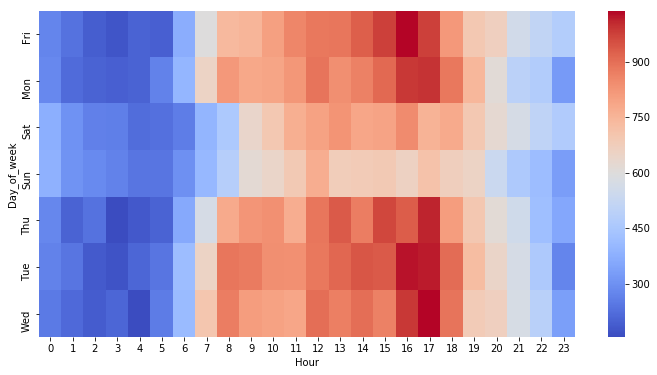### Create a Clustermap using this DataFrame.

``````sns.clustermap(dayHour,cmap='viridis')
``````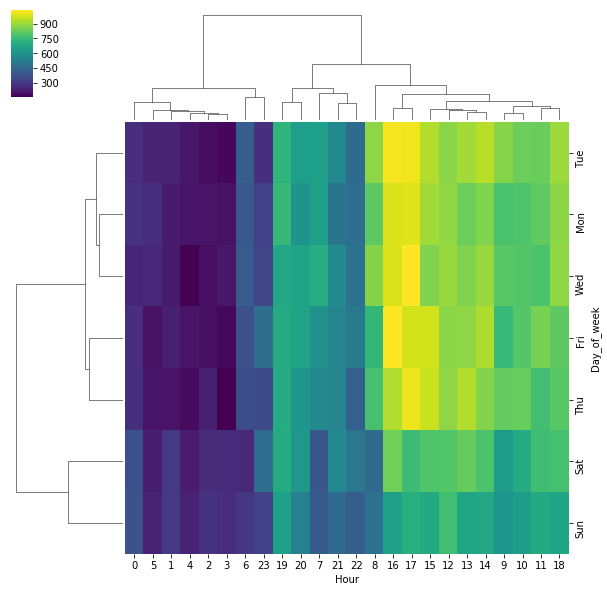Repeat these same plots and operations,

for a DataFrame that shows the Month as the column.

``````dayMonth = df.groupby(by=['Day_of_week','Month']).count()['Reason'].unstack()
``````

### Create a HeatMap by Month column

``````plt.figure(figsize=(12,6))
sns.heatmap(dayMonth, cmap='coolwarm')
``````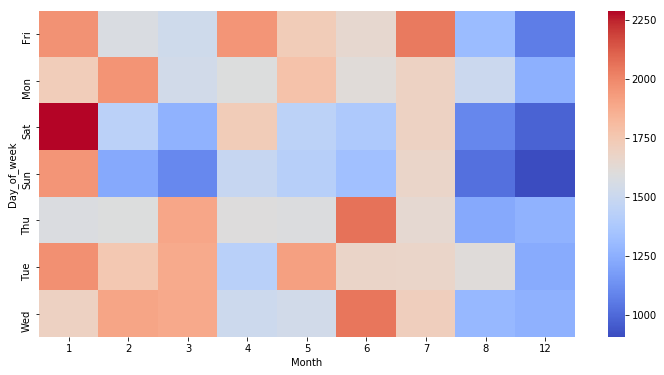### Create a Clustermap by Month column

``````sns.clustermap(dayMonth,cmap='viridis')
``````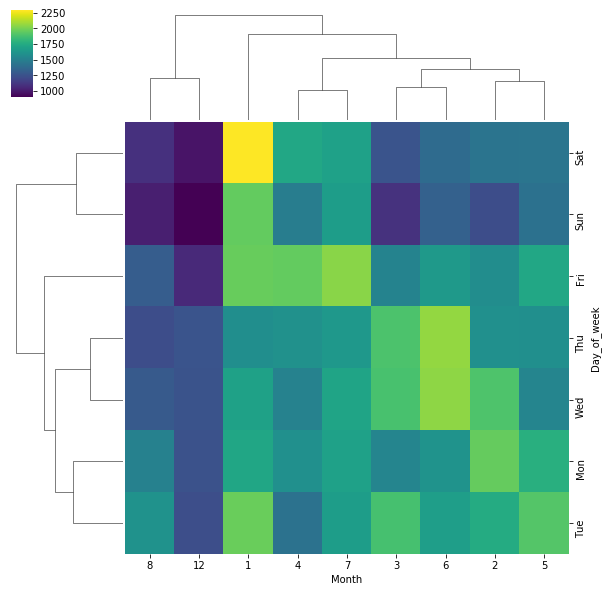So that was the fourth week.. 🔏

https://raw.githubusercontent.com/4bic/4bic.github.io/master/notebooks/911_Project/output_15_1.png